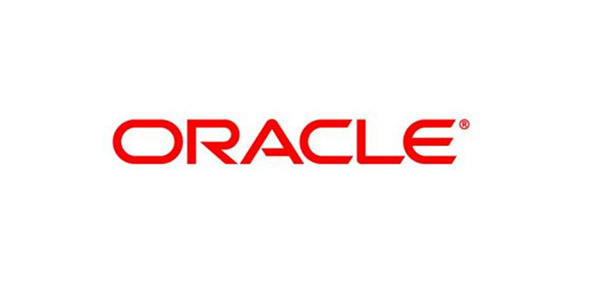# Oracle Test1

12 Questions | Total Attempts: 615SettingsThis is a sample test

• 1.
Evaluate the set of SQLst:   CREATE TABLE dept (deptno NUMBER(2), dname VARCNAR2(14),                                                1oc VARCNAR2 (13)); ROLLBACK; DESCRIBE DEPT        What is true about the set?
• A.

The DESCRIBE DEPT statement displays the structure of the DEPT table.

• B.

The ROLLBACK statement frees the storage space occupies by the DEPT table.

• C.

The DESCRIBE DEPT statement returns an error ORA-04043: object DEPT does not exist.

• D.

The DESCRIBE DEPT statement displays the structure of the DEPT table only if there is a COMMIT statement introduced before the ROLLBACK statement.

• 2.
Evaluate the SQL statement DROP TABLE DEPT:Which four statements are true of the SQL statement?
• A.

You cannot roll back this statement.

• B.

All pending transactions are committed

• C.

All views based on the DEPT table are deleted

• D.

All data in the table is deleted, and the table structure is also deleted

• E.

All data in the table is deleted, but the structure of the table is retained.

• 3.
Which line is incorrect in this query? 1. CREATE TABLE ACTORS( 2. ACTOR_ID NUMBER NOT NULL 3. ,NAME VARCHAR2(32) NOT NULL 4. ,GENDER NUMBER DEFAULT 'M' NOT NULL 5. ,TYPECAST VARCHAR2(64) NULL);
• A.

4

• B.

2

• C.

5

• D.

4,5

• E.

2,5

• 4.
Which of these statements are true with respect to datatypes?
• A.

. NUMBER and FLOAT can be a maximum of 38 bytes.

• B.

The DATE datatype stores date values with a timestamp

• C.

CHAR and VARCHAR2 default to 1 byte if no length is defined.

• D.

Both VARCHAR2 and CHAR can exceed 2,000 bytes.

• E.

VARCHAR2 can be a maximum size of 4,000 bytes; CHAR, 2,000 bytes

• 5.
Which of these datatypes are valid?
• A.

NUMBER(10,2)

• B.

NUMBER(4,5)

• C.

FLOAT(122,2)

• D.

DATE(8)

• E.

CHAR(2500)

• 6.
A datatype is to be forcibly restricted to contain a number with specific restrictions. The number can be a whole number. The number can also contain three digits to the left of the decimal and two digits to the right of the decimal. What is its correct definition?
• A.

NUMBER

• B.

FLOAT

• C.

NUMBER(3,2)

• D.

NUMBER(2,3)

• E.

None of the above

• 7.
Will an error be caused by this CREATE TABLE command? If so, what will cause the error? CREATE TABLE TEST(A FLOAT, B CHAR, C VARCHAR2, D NUMBER , E DATE);
• A.

No error will be returned.

• B.

Yes. The table name TEST is invalid.

• C.

Yes. All columns but the date column must have declared sizes.

• D.

Yes. The length of the VARCHAR2 variable must be defined.

• E.

Yes. The CHAR column must have a declared size.

• 8.
Assuming a table is defined by the CREATE TABLE statement CREATE TABLE TEST(A NUMBER(8,3)); which of these INSERT commands will add a row to the table?
• A.

INSERT INTO TEST VALUES(10000.234);

• B.

INSERT INTO TEST VALUES(1000.2345);

• C.

INSERT INTO TEST VALUES(10000.2345);

• D.

INSERT INTO TEST VALUES(100000.2345);

• E.

INSERT INTO TEST VALUES(100000.24);

• 9.
• A.

DML commands execute an implicit rollback.

• B.

DML commands require an explicit COMMIT to be rolled back.

• C.

DDL commands can be undone.

• D.

DDL commands change data in tables.

• E.

None of the above.

• 10.
Given the table shown, select the INSERT commands that will cause errors: SQL> DESC TEST Name              Null?    Type ----------------- -------- ------------ ID                NOT NULL NUMBER STR               NOT NULL VARCHAR2(32) DEFAULT 'Empty String'
• A.

INSERT INTO TEST(ID) VALUES(1);

• B.

INSERT INTO TEST VALUES(2, 'This is a string');

• C.

INSERT INTO TEST(ID, STR) VALUES(3, 'This is another string');

• D.

INSERT INTO TEST(STR) VALUES('This is another string still');

• E.

INSERT INTO TEST(ID) VALUES('This is yet another string');

• 11.
Which commands will remove all rows from the table assuming that any filters filter out at least one row?
• A.

TRUNCATE TABLE TEST;

• B.

DELETE FROM TEST WHERE ID = 1;

• C.

TRUNCATE TABLE TEST WHERE ID = 1;

• D.

REMOVE TABLE TEST;

• E.

DELETE FROM TEST;

• 12.
Let's assume that a ROLLBACK command is executed after each of the SQL commands listed below. Which of these commands cause the ROLLBACK command to have no effect?
• A.

INSERT

• B.

CREATE TABLE

• C.

CREATE SYNONYM

• D.

MERGE

• E.

TRUNCATE

Related TopicsBack to top